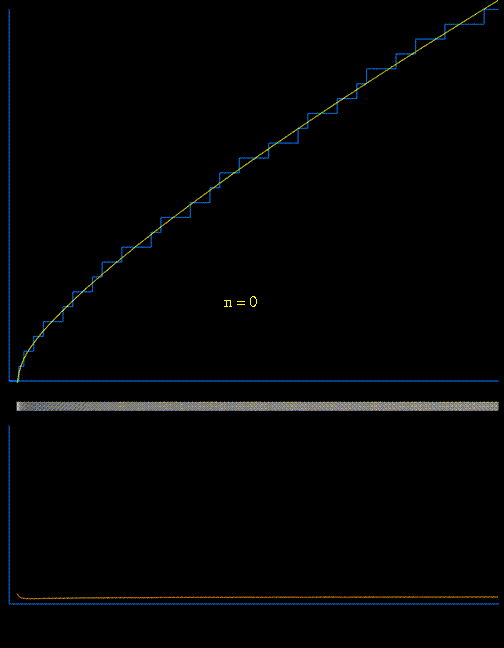# Prime-counting function: Evaluation

According to Riemann (I think) the (exact) prime counting function is given by: $$\pi(x) = \operatorname{R}(x^1) - \sum_{\rho}\operatorname{R}(x^{\rho}) \tag{1}$$ with $$\operatorname{R}(z) = \sum_{n=1}^{\infty} \frac{ \mu (n)}{n} \operatorname{li}(z^{1/n})$$ and $$\rho$$ running over all the zeros of the $$\zeta$$ function.

Why isn't this function used in general to calculate $$\pi(x)$$? Shouldn't it be a great approximation if many zeros of the $$\zeta$$ function are used? Probably nowadays there are many zeros known? Until now I thought $$\pi(x)$$ is a very "hard" function, because the distribution of the primes is quite hard, but this explicit formula does not look that hard.

Thank you.

• We use th zeros of $\zeta(s)$ or evaluating $\pi(x)$, or (it is easier) $\psi(x)$, see this and this – reuns Nov 11 '16 at 3:22

## 2 Answers

I don't know a formal proof of the identity $$\pi(x) = \operatorname{R}(x^1) - \sum_{\rho}\operatorname{R}(x^{\rho}) \tag{1}$$ either but the way this identity was obtained is pretty clear and in fact "hinted" in Riemann's famous paper (cf the german original and english translation linked at the right).
The missing part appears to be proof of the convergence of the series over $\rho$ (supposing the non-trivial zeros sorted by increasing distance to the real axis).

The idea for the derivation (not proof !) is to start with von Mangoldt's proof that (cf Edwards' book p.$48$) : $$\tag{2}f^*(x)=\operatorname{li}(x)-\sum_{\rho} \operatorname{li}(x^{\rho}),\quad(x>1)$$ (notation: $f$ may be replaced by $J$ or $\Pi$ or (confusingly) $\pi$ and $f^*$ appear as $f_0$)
with $\ \displaystyle\operatorname{li}(x):=\int_2^x \frac{dt}{\log\,t}\,$ (Riemann's variant of the logarithmic integral)
and with $\ \displaystyle f^*(x):=\sum_{p^k\le x}^{*}\frac 1k$ (the $^*$ means that the last term $\dfrac 1k$ must be replaced by $\dfrac 12\dfrac 1k\;$ if $p^k=x$)

linked to the prime-counting function $\ \displaystyle\pi^*(x):=\sum_{p\le x}^{*}1\$ by $\ \displaystyle f^*(x)=\sum_{k>0} \frac{\pi^{*}\bigl(x^{1/k}\bigr)}k\qquad (3)$

In fact we need only to apply the Möbius inversion formula $\ \displaystyle\pi^{*}(x):=\sum_{n=1}^{\infty} \frac{\mu(k)}k f^*\bigl(x^{1/k}\bigr)\$ to $(2)$ to get (with questionable convergence...) : $$\tag{4}\boxed{\displaystyle\pi^*(x)=R(x)-\sum_{\rho} R(x^{\rho})},\quad(x>1)$$ Where Riemann's $\,\displaystyle R(x):=\sum_{n=1}^{\infty} \frac{\mu(k)}k \operatorname{li}\bigl(x^{1/k}\bigr)\,$ may be written as a Gram series (this part is an edit of my more complete derivation).

$$-$$

Back to your question "Why isn't this function used in general to calculate $\pi(x)$?". In fact it was used and in a rather successful ways as showed here with some references and the history by Büthe here. Note that $(1)$ had sometimes to be replaced by more effective variants :

Following animation used $(1)$ with increasing number of zeros to generate $\pi(x)$ :(I created it for Matthew Watkins' neat page " 'encoding' of the distribution of prime numbers by the nontrivial zeros of the Riemann zeta function")

It is important to understand that $\;\displaystyle R(x)-\sum_{\rho\ \text{real zero}}R(x^{\rho})$ is the initial smooth part. Without the non trivial zeros you would observe no steps at all! But for the steps to be visible you need enough zeros (see for example p.$12$ of Platt who used nearly $70$ billions zeros to compute $\pi(10^{24})$ and a comparison with the combinatorial methods used by Oliveira e Silva).

• Thanks @ChipHurst even if a better solution could be to edit your answer and search smaller values of $\pi(10^{N})$. Fine continuation anyway, – Raymond Manzoni Nov 11 '16 at 17:24
• Thx for all answers. They are all great and really helped:)! – Kevin Meier Nov 12 '16 at 16:06
• Glad you liked them @Kevin Meier! Excellent continuation, – Raymond Manzoni Nov 12 '16 at 17:18

Borwein, Bradley, and Crandall state on page 249 here that

... it should be the case that, in some appropriate sense $$\pi(x) \sim \text{Ri}(x) - \sum_{\rho} \text{Ri}(x^\rho) \tag{4}$$ with $\text{Ri}$ denoting the Riemann function defi ned: $$\text{Ri}(x) = \sum_{m=1}^\infty \frac{\mu(m)}{m}\text{li}(x^{1/m}). \tag{5}$$ This relation (4) has been called “exact” , yet we could not locate a proof in the literature; such a proof should be nontrivial, as the conditionally convergent series involved are problematic. In any case relation (4) is quite accurate ...

So it appears we don't really know if this relation is indeed true or not.

But as the quote says, it does appear this relation at least provides a good estimate of $\pi$. In fact $\text{Ri}(x)$ alone provides a good estimate of $\pi(x)$. For example, $\pi(10^{20}) = 2220819602560918840$, and here's $\text{Ri}(10^{20})$ evaluated in Mathematica:

In:= Floor[RiemannR[10^20]]

Out= 2220819602556027015


This gives a relative error of about $2.2 \cdot 10^{-12}$, meaning the first 11 digits are correct!

Now how about incorporating the zeros $\rho$? Well they actually seem to make things worse (at least for a 'small' number of zeros). I took the first $14400$ zeros of $\zeta$, to a precision of 30 digits, and got an answer with relative error $3.1 \cdot 10^{-7}$. In fact the more zeros I chose, the worse the relative error became.So to answer your question, I think this formula seems to provide an excellent approximation for $\pi(x)$. However, at the end of the day we'll only be able to get an approximation, not an exact answer.

• Since the series converges, and $\pi(x) \in \mathbb{N}$, we can use it (the $K$ first zeros of $\zeta(s)$) for evaluating $\pi(x)$ exactly at a given $x$ – reuns Nov 11 '16 at 3:24
• @user1952009 Sounds interesting, care to elaborate a bit more? – Chip Hurst Nov 11 '16 at 3:25
• and "So it appears we don't really know if this relation is indeed true or not." of course we know – reuns Nov 11 '16 at 3:25
• @user1952009 I was citing a source which says that. Do you have a reason to believe the identity is true? – Chip Hurst Nov 11 '16 at 3:26
• The RIemann explicit formula is $J(x) = \sum_{p^k < x} \frac{1}{k} = \sum_{n=1}^\infty \frac{\pi(x^{1/n})}{n}$ , $\quad$ $J(x) =li(x) -\sum_\rho li(x^\rho)-\log(2)-\int_x^\infty \frac{dt}{t(t^2-1)\log(t)}dt$ Now by Möbius inversion $\pi(x) = \sum_{n=1}^\infty \mu(n)\frac{J(x^{1/n})}{n}$ by $J(x) = 0$ for $x < 2$ so $\pi(x) = \sum_{n< \log_2(x)} \mu(n)\frac{J(x^{1/n})}{n}$ and you can adapt the Riemann explicit formula accordingly – reuns Nov 11 '16 at 20:33